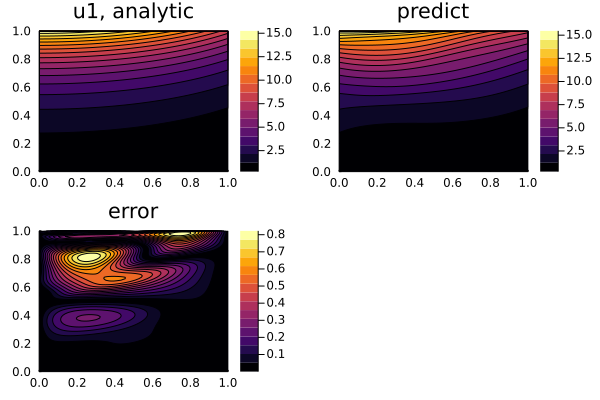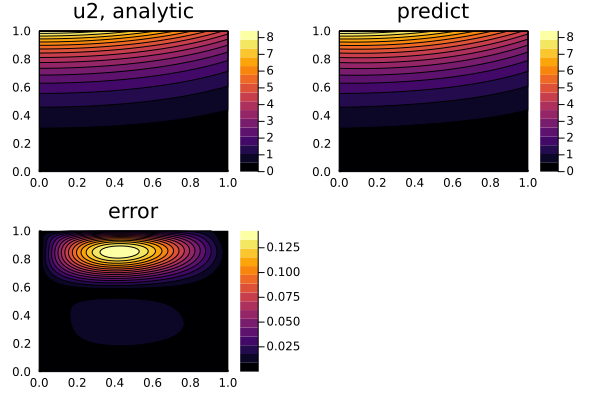# Linear parabolic system of PDEs

We can use NeuralPDE to solve the linear parabolic system of PDEs:

\begin{aligned} \frac{\partial u}{\partial t} &= a * \frac{\partial^2 u}{\partial x^2} + b_1 u + c_1 w \\ \frac{\partial w}{\partial t} &= a * \frac{\partial^2 w}{\partial x^2} + b_2 u + c_2 w \\ \end{aligned}

with initial and boundary conditions:

\begin{aligned} u(0, x) = \frac{b_1 - \lambda_2}{b_2 (\lambda_1 - \lambda_2)} \cdot cos(\frac{x}{a}) - \frac{b_1 - \lambda_1}{b_2 (\lambda_1 - \lambda_2)} \cdot cos(\frac{x}{a}) \\ w(0, x) = 0 \\ u(t, 0) = \frac{b_1 - \lambda_2}{b_2 (\lambda_1 - \lambda_2)} \cdot e^{\lambda_1t} - \frac{b_1 - \lambda_1}{b_2 (\lambda_1 - \lambda_2)} \cdot e^{\lambda_2t} \\ w(t, 0) = \frac{e^{\lambda_1}-e^{\lambda_2}}{\lambda_1 - \lambda_2} \\ u(t, 1) = \frac{b_1 - \lambda_2}{b_2 (\lambda_1 - \lambda_2)} \cdot e^{\lambda_1t} \cdot cos(\frac{x}{a}) - \frac{b_1 - \lambda_1}{b_2 (\lambda_1 - \lambda_2)} \cdot e^{\lambda_2t} * cos(\frac{x}{a}) \\ w(t, 1) = \frac{e^{\lambda_1} cos(\frac{x}{a})-e^{\lambda_2}cos(\frac{x}{a})}{\lambda_1 - \lambda_2} \end{aligned}

with a physics-informed neural network.

using NeuralPDE, Lux, ModelingToolkit, Optimization, OptimizationOptimJL
using Plots
import ModelingToolkit: Interval, infimum, supremum

@parameters t, x
@variables u(..), w(..)
Dxx = Differential(x)^2
Dt = Differential(t)

# Constants
a  = 1
b1 = 4
b2 = 2
c1 = 3
c2 = 1
λ1 = (b1 + c2 + sqrt((b1 + c2)^2 + 4 * (b1 * c2 - b2 * c1))) / 2
λ2 = (b1 + c2 - sqrt((b1 + c2)^2 + 4 * (b1 * c2 - b2 * c1))) / 2

# Analytic solution
θ(t, x) = exp(-t) * cos(x / a)
u_analytic(t, x) = (b1 - λ2) / (b2 * (λ1 - λ2)) * exp(λ1 * t) * θ(t, x) - (b1 - λ1) / (b2 * (λ1 - λ2)) * exp(λ2 * t) * θ(t, x)
w_analytic(t, x) = 1 / (λ1 - λ2) * (exp(λ1 * t) * θ(t, x) - exp(λ2 * t) * θ(t, x))

# Second-order constant-coefficient linear parabolic system
eqs = [Dt(u(x, t)) ~ a * Dxx(u(x, t)) + b1 * u(x, t) + c1 * w(x, t),
Dt(w(x, t)) ~ a * Dxx(w(x, t)) + b2 * u(x, t) + c2 * w(x, t)]

# Boundary conditions
bcs = [u(0, x) ~ u_analytic(0, x),
w(0, x) ~ w_analytic(0, x),
u(t, 0) ~ u_analytic(t, 0),
w(t, 0) ~ w_analytic(t, 0),
u(t, 1) ~ u_analytic(t, 1),
w(t, 1) ~ w_analytic(t, 1)]

# Space and time domains
domains = [x ∈ Interval(0.0, 1.0),
t ∈ Interval(0.0, 1.0)]

# Neural network
input_ = length(domains)
n = 15
chain = [Lux.Chain(Dense(input_, n, Lux.σ), Dense(n, n, Lux.σ), Dense(n, 1)) for _ in 1:2]

discretization = PhysicsInformedNN(chain, strategy)

@named pdesystem = PDESystem(eqs, bcs, domains, [t,x], [u(t,x),w(t,x)])
prob = discretize(pdesystem, discretization)
sym_prob = symbolic_discretize(pdesystem, discretization)

pde_inner_loss_functions = sym_prob.loss_functions.pde_loss_functions
bcs_inner_loss_functions = sym_prob.loss_functions.bc_loss_functions

callback = function (p, l)
println("loss: ", l)
println("pde_losses: ", map(l_ -> l_(p), pde_inner_loss_functions))
println("bcs_losses: ", map(l_ -> l_(p), bcs_inner_loss_functions))
return false
end

res = Optimization.solve(prob, BFGS(); callback = callback, maxiters=5000)

phi = discretization.phi

# Analysis
ts, xs = [infimum(d.domain):0.01:supremum(d.domain) for d in domains]
depvars = [:u,:w]
minimizers_ = [res.u.depvar[depvars[i]] for i in 1:length(chain)]

analytic_sol_func(t,x) = [u_analytic(t, x), w_analytic(t, x)]
u_real  = [[analytic_sol_func(t, x)[i] for t in ts for x in xs] for i in 1:2]
u_predict  = [[phi[i]([t,x], minimizers_[i]) for t in ts  for x in xs] for i in 1:2]
diff_u = [abs.(u_real[i] .- u_predict[i]) for i in 1:2]
for i in 1:2
p1 = plot(ts, xs, u_real[i], linetype=:contourf, title="u$i, analytic"); p2 = plot(ts, xs, u_predict[i], linetype=:contourf, title="predict"); p3 = plot(ts, xs, diff_u[i], linetype=:contourf, title="error"); plot(p1, p2, p3) savefig("sol_u$i")
end